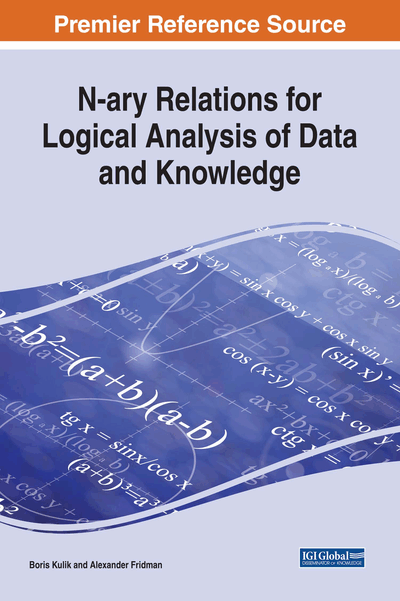# NTA Techniques to Decrease Complexity

DOI: 10.4018/978-1-5225-2782-4.ch005

## Abstract

Matrix properties of NTA objects allow to decrease computational complexity of intellectual procedures as well as to efficiently parallel logical inference algorithms. New structural and statistical classes of CNFs with polynomially identifiable satisfiability properties were discovered in NTA. Consequently, many classes of problems, whose complexity evaluation is theoretically high, e.g., exponential, can in practice be solved in polynomial time, on the average.
Chapter Preview

If a man takes no thought about what is distant, he will find sorrow near at hand. – Confucius

Top

## Orthogonalization

Analyzing above examples of C-systems, it can be seen that some pairs of the C-n-tuples they contain have non-empty intersections. Sometimes, it complicates calculations heavily. For instanc, when it is necessary to calculate the total number of elementary tuples contained in such C-systems, all possible intersections of pairs of C-n-tuples, their triples, etc. must be considered. The same difficulties arise during studies of any metric properties of NTA objects, such as their probabilistic representation. Orthogonalization is deliberately designed to overcome these difficulties.

Furthermore, orthogonalization provides an effective means for reducing complexity of algorithms for converting NTA objects into alternative classes, D-systems into C-systems in particular. The fundamental theorem of orthogonalization was formulated and proved by the Russian logician P.S. Poretsky (1887).

Let us consider the basic relations for orthogonalization used in mathematical logic. A DNF is called an orthogonal one, if every pair of its conjuncts has no common satisfying substitutions.

## Complete Chapter List

Search this Book:
Reset